### DSUM Function – Examples in Excel, VBA, Google Sheets

This Tutorial demonstrates how to use the Excel DSUM Function in Excel to sum records in a database.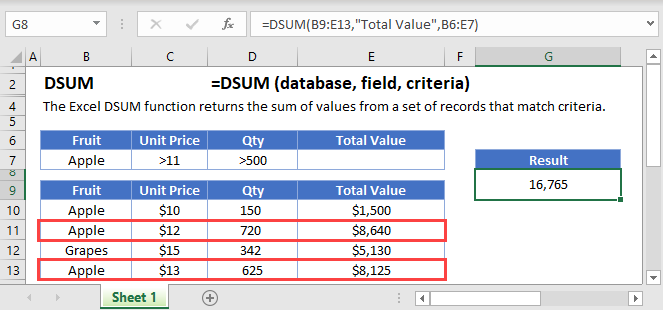## DSUM Function Overview

The DSUM Function Calculates the sum of a field in a database for records that meet certain criteria.

To use the DSUM Excel Worksheet Function, select a cell and type: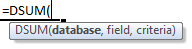(Notice how the formula inputs appear)

## DSUM function Syntax and inputs:

`=DSUM(database,field,criteria)`

database – The database range with field names in the top row.

field – The field within the database to sum. This can either be the field name (ex: “birth date”) or column number (ex: 5).

criteria – The range of cells containing the criteria that indicates which records to sum. Example:

# What is the DSUM Function?

DSUM is one of Excel’s database functions. It returns the sum of a column in a database, after applying a range of filters to the data.

Note that when we say “database” in this context, we just mean a table of data in Excel, that has been organized with column headers.

AutoMacro - VBA Code Generator

## How to Use the DSUM Function

To use the Excel DSUM Function, type the following:

`=DSUM(B7:F19,"Oscars won",B3:F4)`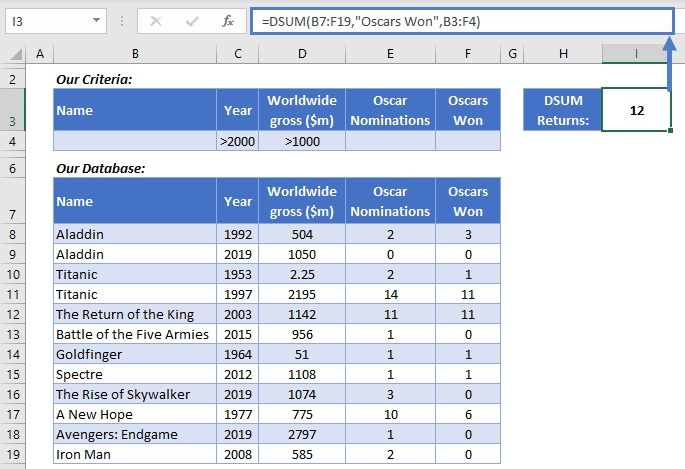We have a database with some movie data. We want to know how many Oscars were won by movies released after the year 2000, that grossed over \$1bn. DSUM can get that information for us.

Here’s how DSUM works:

• The first argument is the database, which we’ve got in cells B7:F19. Note: you must include your column headers in this range.
• The second argument is the column we want to sum – Oscars Won. You can refer to columns by their name in quotations, or by their numerical position (so we could also just put 5 here).
• In the third argument we define the filters we want DSUM to apply before calculating the sum. These are in B3:F4.

Take a closer look at the filters we’ve defined in B3:F4. We’ve got the same headers as the database, and we’ve defined two criteria: a “Year” greater than 2000, and a “Worldwide Gross (\$m)” greater than 1000.

DSUM correctly returns 12 Oscars. Two movies meet these criteria: The Return of the King, doing the monarch’s share of the work with 11 Oscars, and Spectre, which adds 1 more to the total.

## DSUM Sums Numerical Values Only

DSUM will only include rows with numerical data, and will ignore text. For example, if we try summing the “Name” column, DSUM will return 0, because it doesn’t find any numerical data to sum.

`=DSUM(B7:F19,"Name",B3:F4)`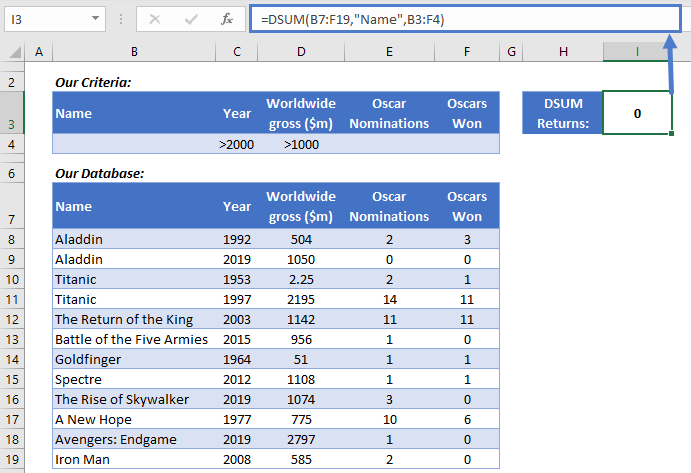## What You Can Use as Criteria

DSUM enables you to use a range of different criteria when filtering your data. Here are a few common examples: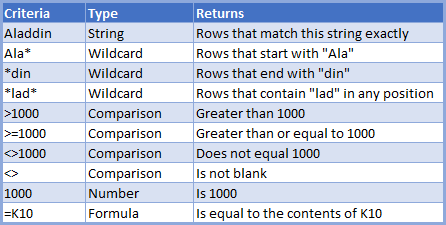## Using Multiple Criteria Rows

When you create your criteria table, you can use more than one row if you need to.

If you do use more than one row, DSUM will use “OR” logic – it will include data that matches any of your criteria rows.

Here’s an example:

`=DSUM(B8:F20, 5,B3:C5)`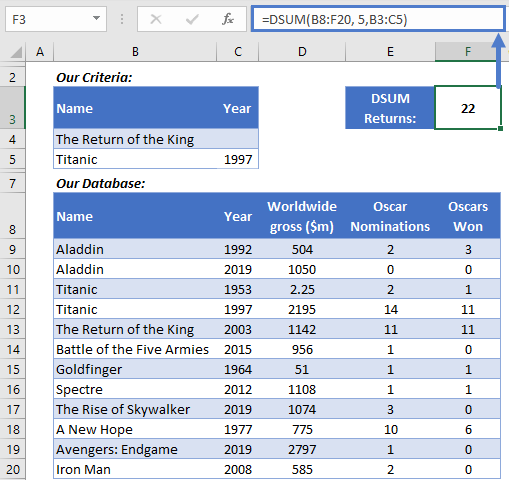We want to know how many Oscars 1997’s Titanic and 2003’s The Return of the King won in total. Since there are two movies called “Titanic” in the database, we’ve also specified the year in our criteria table.

Note also, we’ve only used the two columns we want to filter on in our criteria table – you don’t need to include them all.

DSUM returns 22 – 11 for each movie.

## Use SUM When You Don’t Need to Filter the Data

DSUM is a powerful tool when you need to filter the data in complex ways. But if you don’t need to use filters, use SUM instead.

You use SUM like this:

`=SUM(C3:C14)`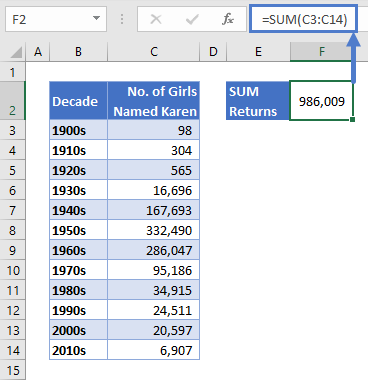As you can see here, with SUM you simply define the range containing your data, and it will return the sum of any numerical cells within that range.

Learn more on the main Excel SUM Function<<link>> page.

## DSUM in Google Sheets

The DSUM Function works exactly the same in Google Sheets as in Excel: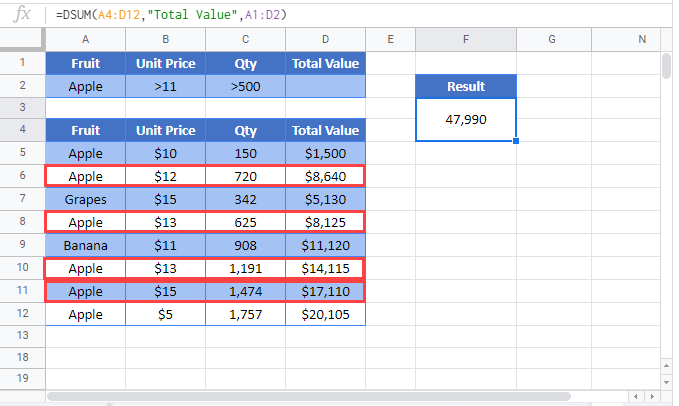## Additional Notes

Use the DSUM Function to add database records that meet certain criteria.

First, indicate the array that contains the database. IMPORTANT: The first row of the database must contain field names.

Next, indicate which field within the database to sum. Either enter the field name, enclosed by quotations (ex: “Age”) or enter a number corresponding with the field’s position within the database (ex: 2 for second column).

Last, reference the range of cells that contains the criteria to use. The Criteria must have at least one field name with a condition below it. You can enter more fields in separate columns to add more conditions.

## DSUM Examples in VBA

You can also use the DSUM function in VBA. Type:
`application.worksheetfunction.dsum(database,field,criteria)`
For the function arguments (database, etc.), you can either enter them directly into the function, or define variables to use instead.

Return to the List of all Functions in Excel

## Excel Practice Worksheet

Practice Excel functions and formulas with our 100% free practice worksheets!

• Automatically Graded Exercises
• Learn Excel, Inside Excel!